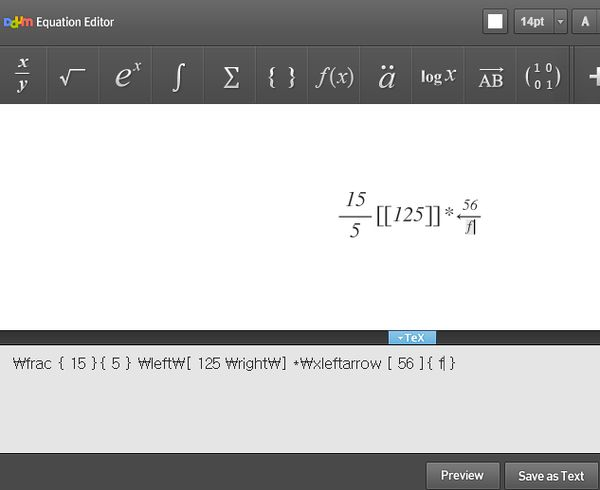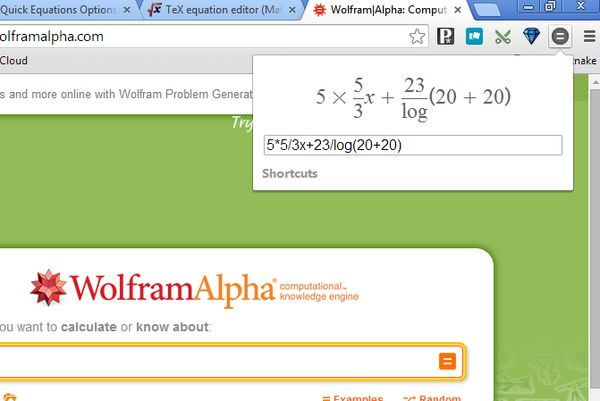Editor Ratings:
User Ratings:
[Total: 1 Average: 5]

Here’s a list of 4 equation editor extensions for Google Chrome which you can use to easily edit equations using Chrome. Writing equations isn’t as easy as it might seem, because there’s a lot of non-standard symbols used in math that aren’t easy to jot down using the keyboard. To create mathematical equations you need to have special editor, kind of like the ones that we’re about to talk about.

Let’s see what available in Chrome webstore.

##(adsbygoogle = window.adsbygoogle || []).push({});

With Daum you get a very advanced graphical equation editor where at the top you have a toolbar with all the standard types of math symbols for creating formulas.

As you’re writing equations, they are converted at the bottom into Tex syntax, if you wanna copy it into a mathematical Tex powered editor. Created equations can be exported as a text document, PNG image or saved directly to your Google Drive account.

##TeX Equation Editor is another math editor for Chrome that focuses on TeX syntax, like you can see from the image above.

Using the extension is easy. Create your mathematical equation by selecting math symbols from the top panel. As you’re selecting them the TeX syntax changes in the main editor down below. Change values directly in the editor. Equation preview updates right away underneath the main editor. Created equations and formulas can be downloaded as an image.

##(adsbygoogle = window.adsbygoogle || []).push({});

Quick Equations adds a math equation editor to the Chrome’s top right corner toolbar. Editor will pop-up when you left click on its icon.

With this equation editor extension you’ll be able to quickly type out an equation and see how it actually looks like before submitting it to Wolfram Alpha, for example. Type out the equation you need to solve, and the preview field on top of the input box will change in real time and give you preview of how the equation really looks like.

Get Quick Equations.

##If you ever came across a TeX expression on a website and wanted to see how it would look like rendered then this extension is just the thing you need.

Technically speaking this isn’t an equation editor, but you can use in situations when a text input field doesn’t have a preview option for TeX syntax and you can’t be sure how the equation that you type will look like. By installing this extension you’ll be able to easily preview how a TeX math equation looks like rendered, right on the website.

Get Tex Renderer.

## Conclusion

In our expert opinion the first two extensions from the list, Daum Equation Editor and TeX Equation Editor are the most useful math equation editor extensions for Chrome that you’ll be able to find in Chrome store. They have easy to understand, point-and-click approach to creating equations, while at the same time allowing advanced users to edit the TeX syntax by hand. Try them all and let us know what you think in the comments down below.

 Editor Ratings: User Ratings:[Total: 1 Average: 5] Works With: Google Chrome Free/Paid: Free